NCERT Solutions for Class 10 Maths Chapter 5 Arithmetic Progressions Ex 5.3 are part of NCERT Solutions for Class 10 Maths. Here we have given NCERT Solutions for Class 10 Maths Chapter 5 Arithmetic Progressions Ex 5.3.

 Board CBSE Textbook NCERT Class Class 10 Subject Maths Chapter Chapter 5 Chapter Name Arithmetic Progressions Exercise Ex 5.3 Number of Questions Solved 20 Category NCERT Solutions

## NCERT Solutions for Class 10 Maths Chapter 5 Arithmetic Progressions Ex 5.3

Question 1.
Find the sum of the following APs:
(i) 2, 7, 12,…… to 10 terms.
(ii) -37, -33, -29, …… to 12 terms.
(iii) 0.6, 1.7, 2.8, ……, to 100 terms.
(iv) $$\frac { 1 }{ 15 }$$, $$\frac { 1 }{ 12 }$$, $$\frac { 1 }{ 10 }$$, …….., to 11 terms.
Solution: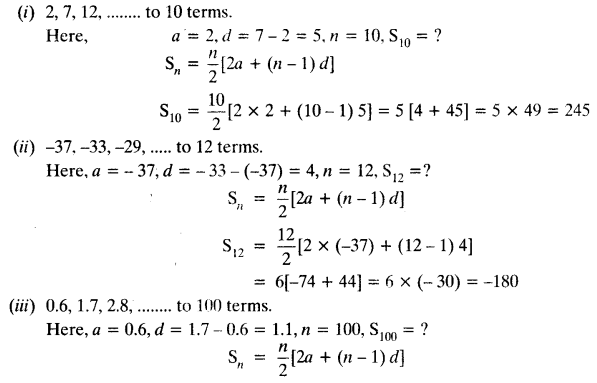Question 2.
Find the sums given below:
(i) 7 + 10$$\frac { 1 }{ 2 }$$ + 14 + … + 84
(ii) 34 + 32 + 30 + … + 10
(iii) -5 + (-8) + (-11) + ….. + (-230)
Solution: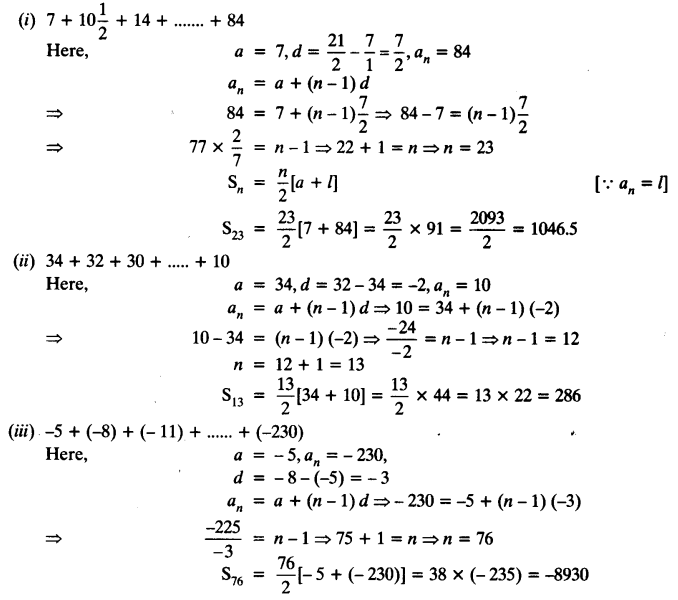Question 3.
In an AP:
(i) given a = 5, d = 3, an = 50, find n and Sn.
(ii) given a = 7, a13 = 35, find d and S13.
(iii) given a12 = 37, d = 3, find a and S12.
(iv) given a3 = -15, S10 = 125, find d and a10.
(v) given d = 5, S9 = 75, find a and a9.
(vi) given a = 2, d = 8, Sn = 90, find n and an.
(vii) given a = 8, an = 62, Sn = 210, find n and d.
(viii) given an = 4, d = 2, Sn = -14, find n and a.
(ix) given a = 3, n = 8, S = 192, find d.
(x) given l = 28, S = 144, and there are total 9 terms. Find a.
Solution: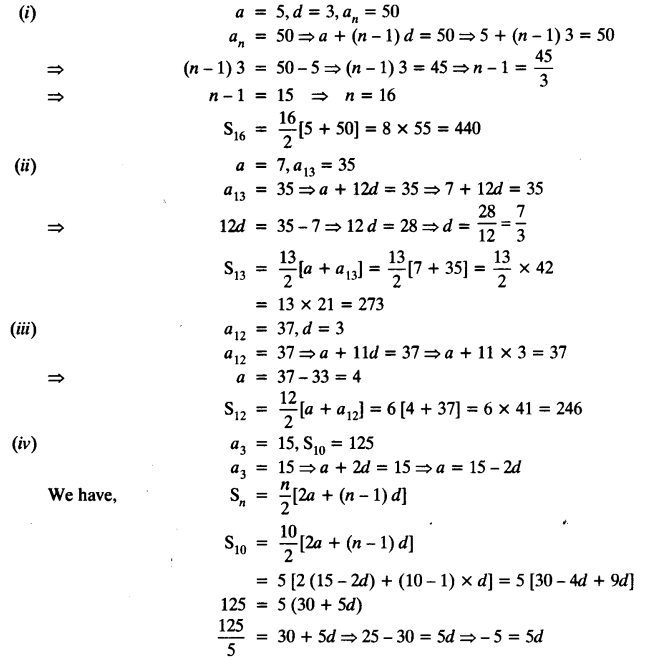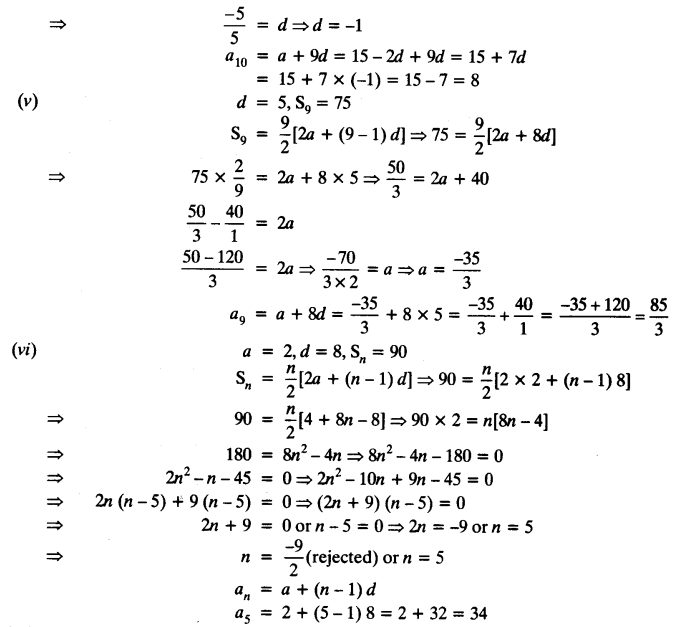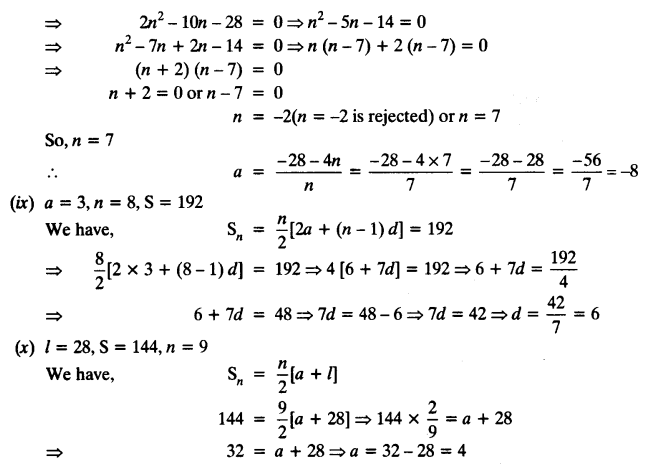Question 4.
How many terms of AP: 9, 17, 25, … must be taken to give a sum of 636?
Solution:Question 5.
The first term of an AP is 5, the last term is 45 and the sum is 400. Find the number of terms and the common difference.
Solution: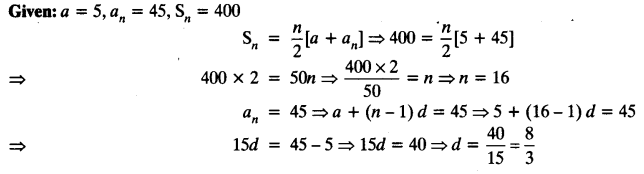Question 6.
The first and the last terms of an AP are 17 and 350 respectively. If the common difference is 9, how many terms are there and what is their sum?
Solution:Question 7.
Find the sum of first 22 terms of an AP in which d = 7 and 22nd term is 149.
Solution: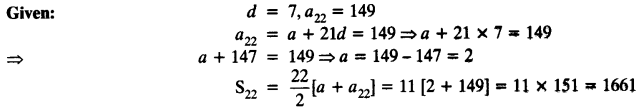Question 8.
Find the sum of first 51 terms of an AP whose second and third terms are 14 and 18 respectively.
Solution:Question 9.
If the sum of first 7 terms of an AP is 49 and that of 17 terms is 289, find the sum of first n terms.
Solution: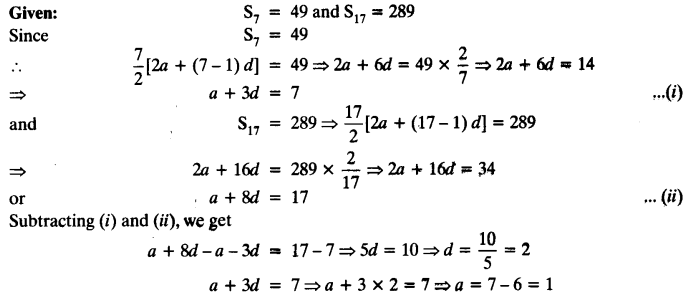Question 10.
Show that a1, a2, ……. an,…… form an AP where an is defined as below:
(i) an = 3 + 4n
(ii) an = 9 – 5n
Also find the sum of the first 15 terms in each case.
Solution: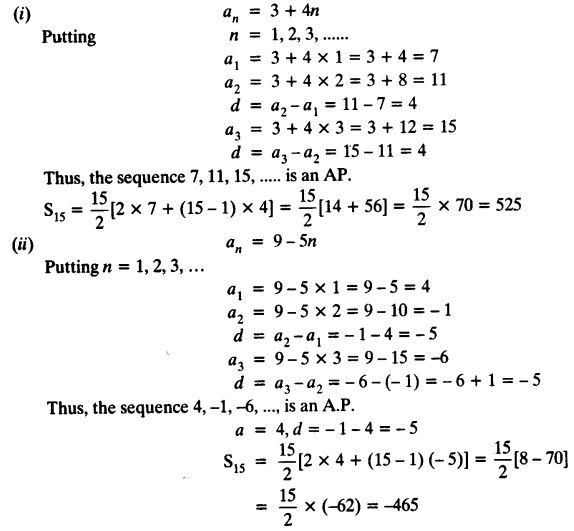Question 11.
If the sum of the first n terms of an AP is 4n – n2, what is the first term (that is S1)? What is the sum of first two terms? What is the second term? Similarly, find the 3rd, the 10th and the nth terms.
Solution:Question 12.
Find the sum of the first 40 positive integers divisible by 6.
Solution: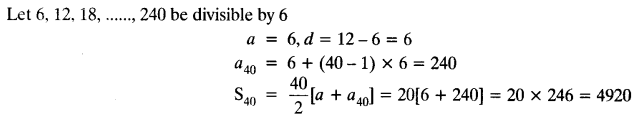Question 13.
Find the sum of the first 15 multiples of 8.
Solution: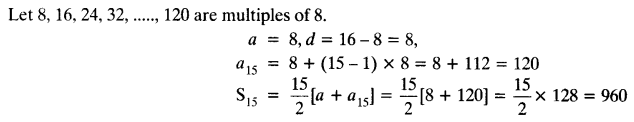Question 14.
Find the sum of the odd numbers between 0 and 50.
Solution:
Let odd numbers between 0 and 50 be 1, 3, 5, 7,…….., 49.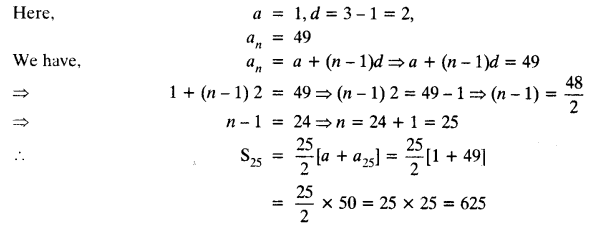Question 15.
A contract on construction job specifies a penalty for delay of completion beyond a certain date as follows:
₹ 200 for the first day, ₹ 250 for the second day, ₹ 300 for the third day, etc. the penalty for each succeeding day being ₹ 50 more than for the preceding day. How much money the contractor has to pay as penalty, if he has delayed the work by 30 days?
Solution:Question 16.
A sum of ₹ 700 is to be used to give seven cash prizes to students of a school for their overall academic performance. If each prize is ₹ 20 less than its preceding prize, find the value of each of the prizes.
Solution:
Let 1st prize be of ₹ a
2nd prize be ₹ (a – 20) and
3rd prize be ₹ (a – 20 – 20) = ₹ (a – 40)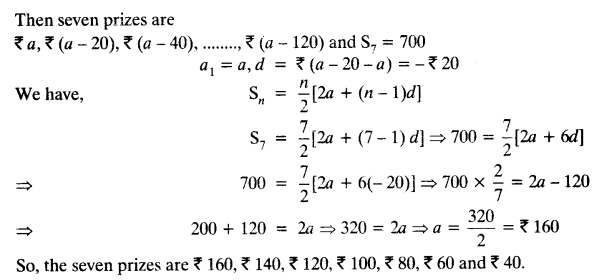Question 17.
In a school, students thought of planting trees in and around the school to reduce air pollution. It was decided that the number of trees, that each section of each class will plant, will be the same as the class, in which they are studying, eg. a section of Class I will plant 1 tree, a section of Class II will plant 2 trees and so on till Class XII. There are three sections of each class. How many trees will be planted by the students?
Solution:
Let the trees be planted 1, 2, 3, 4, 5 , …… 12
Here, a = 1, d = 1, n = 12
Total number of trees planted by each section
S12 = $$\frac { 12 }{ 2 }$$ [2a + (n – 1) d] = 6 [2 x 1 + (12 – 1) x 1]
= 6 [2 + 11] = 6 x 13 = 78
Total number of trees planted by 3 sections = 78 x 3 = 234

Question 18.
A spiral is made up of successive semicircles, with centres alternately at A and B, starting with centre at A, of radii 0.5 cm, 1.0 cm, 1.5 cm, 2.0 cm,… as shown in figure. What is the total length of such a spiral made up of thirteen consecutive semicircles?
(Take π = $$\frac { 22 }{ 7 }$$)
[Hint: Length of successive semicircles is l1, l2, l3, l4, … with centres at A, B, respectively.]Solution: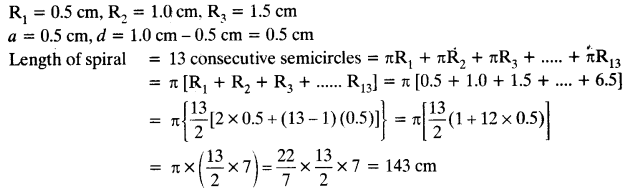Question 19.
200 logs are stacked in the following manner 20 logs in the bottom row, 19 in the next row, 18 in the row next to it and so on (see Figure). In how many rows are the 200 logs placed and how many logs are in the top row?Solution: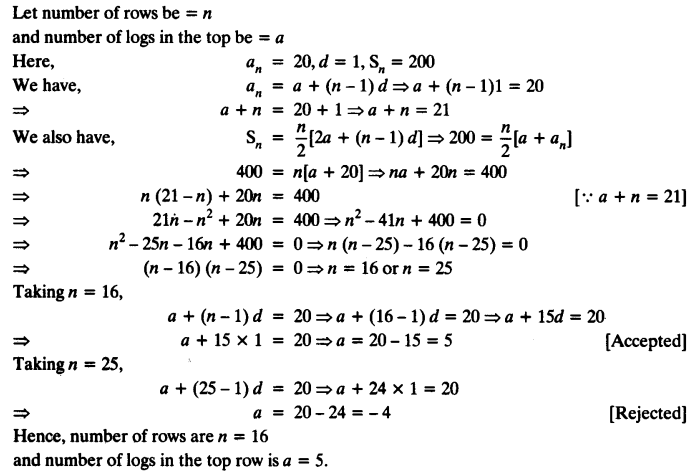Question 20.
In a potato race, a bucket is placed at the starting point, which is 5 m from the first potato, and the other potatoes are placed 3 m apart in a straight line. There are ten potatoes in the line (see Fig.) A competitor starts from the bucket, picks up the nearest potato, runs back with it, drops it in the bucket, runs back to pick up the next potato, runs to the bucket to drop it in, and she continues in the same way until all the potatoes are in the bucket. What is the total distance the competitor has to run?
[Hint: To pick up the first potato and the second potato, the total distance (in metres) run by a competitor is 2 x 5 + 2 x (5 + 3)]Solution:
Distance between the first potato and the bucket = 5 m
Distance between next 2 potatoes = 3 m each
So, series is 5 m, 8 m, 11 m,
Here, a = 5 m, d = (8 – 5) m = 3 m
Total distance travelled for 10 potatoes = 2 [5 + 8 + 11 + …….. + 10 terms]
= 2[$$\frac { 10 }{ 2 }$${2 x 5 + (10 – 1) 3}]
= 2[5{10 + 27}] = 2(37 x 5) = 37 x 10 = 370 m.

We hope the NCERT Solutions for Class 10 Maths Chapter 5 Arithmetic Progressions Ex 5.3, help you. If you have any query regarding NCERT Solutions for Class 10 Maths Chapter 5 Arithmetic Progressions Ex 5.3, drop a comment below and we will get back to you at the earliest.